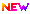Home > Operation Research calculators > Integer Simplex method (Gomory's cutting plane method) calculator

 ** check different types of Integer simplex method (gomory's cutting plane method) examples Algorithm and examplesMethod 1. Simplex method (BigM method) 2. TwoPhase method 3. Dual simplex method 4. Integer simplex method 5. Graphical method 6. Primal to Dual 7. Branch and Bound method 8. 0-1 Integer programming problem 9. Revised Simplex method Solve the Linear programming problem using Integer simplex method (gomory's cutting plane method) calculator Type your linear programming problem OR Total Variables :   Total Constraints : Click On Generate Mode : Decimal Fraction Zj-Cj (display in steps) Alternate Solution (if exists) Artificial Column Remove Subtraction Steps max Z = x1 + x2subject to3x1 + 2x2 <= 5x2 <= 2and x1,x2 non-negative integersmax Z = 2x1 + 20x2 - 10x3subject to2x1 + 20x2 + 4x3 <= 156x1 + 20x2 + 4x3 = 20and x1,x2,x3 non-negative integersmax Z = 3x1 + 12x2subject to2x1 + 4x2 <= 75x1 + 3x2 <= 15and x1,x2 non-negative integersmax Z = -3x1 + x2 + 3x3subject to-x1 + 2x2 + x3 <= 42x2 - 3/2x3 <= 1x1 - 3x2 + 2x3 <= 3and x1,x2 >= 0 and x3 non-negative integersmax Z = x1 + x2subject to3x1 + 2x2 <= 5x2 <= 2and x2 >= 0 and x1 non-negative integers

SolutionHelp